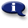# .

## Syllabus Information

Fall 2022
Dec 03,2023Use this page to maintain syllabus information, learning objectives, required materials, and technical requirements for the course.

Syllabus Information
MTH 010 - Whole Numbers, Fractions, Decimals
Associated Term: Fall 2022
Learning Objectives: Upon successful completion of this course, the student will be able to:
1. Add, subtract, multiply, and divide whole numbers
2. Identify characteristics of even, odd, prime, and composite numbers
3. Solve real world application problems using whole numbers
4. Order whole numbers using < and >
5. List factors and multiples of a given number
6. Compute problems using the order of operations
7. Use math vocabulary
8. Compute area and perimeter of rectangles using whole numbers
9. Add, subtract, multiply, and divide fractions with like and unlike denominators
10. Reduce fractions
11. Compare fractions using <, > or =
12. Convert fractions to decimals
13. Solve real world problems using fractions
14. Compute area and perimeter of rectangles using fractions
15. Use vocabulary of fraction terms
16. Add, subtract, multiply, and divide using decimals
17. Identify place value in decimal numbers
18. Compare decimals using <, > or =
19. Convert decimals to fractions
20. Solve real world application problems using decimals
21. Compute area and perimeter of rectangles using decimals
22. Selects appropriate math study strategies
23. Monitors and evaluates personal confidence progress
24. Utilizes appropriate math resources
Required Materials:
Technical Requirements: